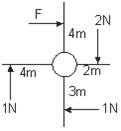# ICSE Worksheet for chapter-2 Force class 10

#### Worksheet For class 10

This page is prepared by the Academic team of Physics Wallah which consists of ICSE Board Worksheet for Class 10 Physics. Students of Class 10 Physics can get a free Worksheet for Class 10 Physics in PDF format prepared as per the newest syllabus and examination pattern in your schools.

Standard 10 students can practice questions and answers which are given here for Physics in Grade 10 that will help them to improve their knowledge of all important chapters and their topics. Students can also download free pdf of Class 10 Physics Notes prepared by teachers and solve important problems provided here with solutions on daily basis to get more scores in school exams and tests.

If any students need to take the online test to check their concepts or undertstanding then they can visit Physics Quiz for Class 10.

## Summary

• Moment of a force and Equilibrium, Translational and Rotational motions, Units of torque
• Equilibrium of bodies, Kinds of equilibrium –Static and Dynamic, Principle of moments
• Centre of gravity, Uniform circular motion, Centripetal force, Centrifugal force

### Section 1 - Objective

Q1. …………. is the tendency of a force to rotate an object about an axis.

1. Mass
2. Inertia
3. Torque
4. Centre of gravity

Q2. S.I unit if couple is

1. N-m
2. dyne-m
3. N-m/s2
4. N

Q3. C.G of a triangular lamina is at its.

1. Centre
2. Outside
3. Centroid
4. NOT

Q4. The point through which all the weight appears to act for any orientation of the objects is

1. Velocity point
2. Centre of gravity
3. Torque
4. NOT

Q5. The coordinates of edges of a square are O (0, 0), A(0,4),B (4,4),C (4,0) respectively, the center of gravity of square is at coordinates

1. (0, 2)
2. (2, 0)
3. (2, 2)
4. (0, 0)

Q6. A windmill is pushed by four external forces as shown. Calculate the force F required to make the windmill standstill.1. 2N
2. 4N
3. 6N
4. NOT

Q7. When a particle moves with constant speed in circular path, its motion is said to be

1. Linear motion
2. Circular motion
3. Both a & b
4. Not

Q8. Uniform circular motion is an example of

1. Accelerated motion
2. Unaccelerated motion
3. Linear motion
4. NOT

Q9. The principle involved in construction of beam balance is

1. Principle of moments
2. Principle of inertia
3. Principle of velocity
4. Principle of superposition

Q10. Which of the following quantity remains constant in U.C.M?

1. Velocity
2. Acceleration
3. Speed
4. Force

Q11. A particle moves in a circle of radius 20cm with a linear speed of 10 m/s. Find its angular velocity?

Q12. A particle travels in a circle of radius 20 cm at a speed that uniformly increases. If the speed changes from 5 m/s to 6 m/s in 2s. Find the angular acceleration.

### Section 2 - Objective

Q1. Define U.C.M with any 3 examples.

Q2. Distinguish between centripetal and centrifugal force.

Q3. Differentiate between Uniform circular motion and /uniform linear motion.

Q4. Two forces each of magnitude 2N act vertically upwards and downwards respectively on two ends of uniform rod of length 1 m which is pivoted at its center. Draw the diagram of the arrangement and determine the resultant moment of force about the mid-point of the rod.

Q5. State the condition when force produces a) Translational motion b) rotational motion.

Q6. Define Centre of gravity.

Q7. What do you mean by equilibrium of a body? State the conditions of equilibrium.

Q8. What is meant by moment of force? If the moment of force is assigned a negative sign then will the turning tendency of the force be clockwise or anticlockwise?

Q9. A uniform metre scale can be balanced at the 70cm mark when a mass of 0.05Kg is hung from the 94cm mark.

1. Draw the diagram of the arrangement
2. Find the mass of the metre scale

### Solutions

1. 3
2. 1
3. 3
4. 2
5. 3
6. 4
7. 2
8. 1
9. 1
10. 3
11. 2
12. 4

#### Subjective Problems

Q8. Clockwise

Q9. 2. m = 0.06kg